Complex Sinusoids as Circular Motion Next  |  Prev  |  Up  |  Top  |  Index  |  JOS Index  |  JOS Pubs  |  JOS Home  |  Search

Plotting Complex Sinusoids as Circular Motion

Figure 1.8 shows Euler's relation graphically as it applies to sinusoids. A point traveling with uniform velocity around a circle with radius 1 may be represented by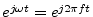in the complex plane, where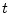is time and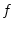is the number of revolutions per second. The projection of this motion onto the horizontal (real) axis is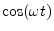, and the projection onto the vertical (imaginary) axis is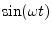. For discrete-time circular motion, replaceby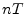to get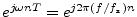which may be interpreted as a point which jumps an arc length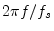radians along the circle each sampling instant.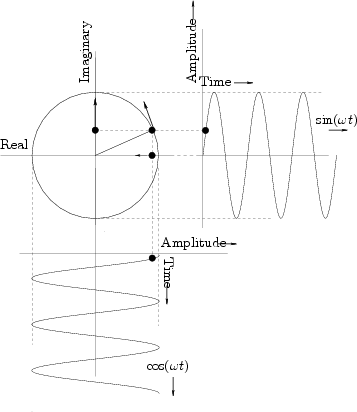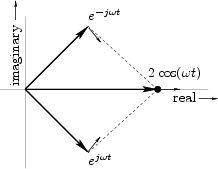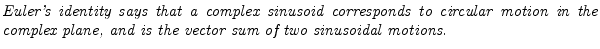For circular motion to ensue, the sinusoidal motions must be at the same frequency, one-quarter cycle out of phase, and perpendicular (orthogonal) to each other. (With phase differences other than one-quarter cycle, the motion is generally elliptical.)

The converse of this is also illuminating. Take the usual circular motion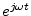which spins counterclockwise along the unit circle asincreases, and add to it a similar but clockwise circular motion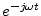. This is shown in Fig.1.9. Next apply Euler's identity to get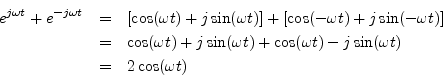Thus,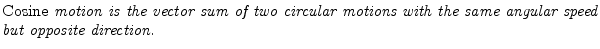This statement is a graphical or geometric interpretation of Eq. (1.11). A similar derivation (subtracting instead of adding) gives the sine identity Eq. (1.12).

We calla positive-frequency sinusoidal component when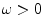, andis the corresponding negative-frequency component. Note that both sine and cosine signals have equal-amplitude positive- and negative-frequency components (see also [83,53]). This happens to be true of every real signal (i.e., non-complex). To see this, recall that every signal can be represented as a sum of complex sinusoids at various frequencies (its Fourier expansion). For the signal to be real, every positive-frequency complex sinusoid must be summed with a negative-frequency sinusoid of equal amplitude. In other words, any counterclockwise circular motion must be matched by an equal and opposite clockwise circular motion in order that the imaginary parts always cancel to yield a real signal (see Fig.1.9). Thus, a real signal always has a magnitude spectrum which is symmetric about 0 Hz. Fourier symmetries such as this are developed more completely in .

Next  |  Prev  |  Up  |  Top  |  Index  |  JOS Index  |  JOS Pubs  |  JOS Home  |  Search

[How to cite this work] [Order a printed hardcopy]